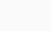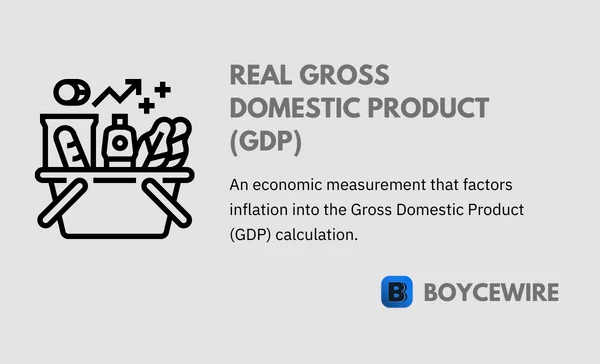# Real GDP: Definition & How to Calculate

Table of Contents# Real Gross Domestic Product (Real GDP): Definition & How to Calculate## What is Real Gross Domestic Product (Real GDP)?

Real Gross Domestic Product (Real GDP) is Gross Domestic Product (GDP) that accounts for inflation or deflation. In other words, it disregards the impact that prices play in economic growth and allows us to compare the quantity of goods produced rather than the value.

By accounting for inflation, real GDP is able to identify the ‘real’ growth in the economy. This adjusts for price fluctuations and looks at purely the number of goods.

##### Key Points
1. Real Gross Domestic Product (Real GDP) is Gross Domestic Product that accounts for inflation or deflation.
2. It allows us to better compare economic growth year on year as it accounts for fluctuations in the supply of money – allowing us to compare the quantity and value of goods produced each year.
3. We can calculate the economic measurement by dividing nominal GDP over a GDP deflator which measures inflation.

If we take an example, a Burger costs \$10 in Year 1. In Year 2, it may have risen to \$11 – indicating an inflation rate of 10 percent. This extra \$1 is included in GDP, but does not mean that more goods or services have been created. In both Year 1 and Year 2 – one burger has been manufactured, which it accounts for.

Real GDP considers inflation and removes it from the economic output calculation, which gives an accurate representation of economic growth year on year. So in this case, the increase in \$1 for the burger is not considered – meaning real economic output would remain at \$10 as far as the burger is concerned. This allows us to be able to better identify year on year increases in quantity as we can disregard increases in price.

## Why Real GDP is used

As GDP calculates the amount of goods and service produced in a country, it is important to see how this grows over time. For instance, everything in the economy could increase in price by 10 percent. This would then show a 10 percent growth in economic output, but this does not represent the physical goods produced.

We measure GDP using numbers and currency, usually because the information is more easily digested. For instance, it is easier for us to say US GDP is worth \$20 trillion; rather than 1 million Fords, 2 billion loaves of bread, 500 million eggs, so on and so forth. However, whilst this is easier for us to determine the value of economic output – it does not help us determine the quantity. By removing inflation, we are able to get a better understanding of how much quantity has increased, but on a value basis.

#### “By factoring in inflation, Real GDP gives economists a more accurate look at how well an economy is performing.”

We use Real GDP in order to get an accurate measure of the economy. This is because nominal GDP measures the value of the economic output, but this doesn’t create a full and accurate picture of how the economy is performing. For instance, Venezuela experienced rapid levels of inflation through the early 21st century – sometimes reaching over 1,000 percent. In such a scenario, it is obvious that the economy is not truly growing at 1,000 percent a year.

To conclude, Real GDP adjusts the nation’s economic output so that it considers the GDP in terms of volume growth as opposed to value growth. This allows policymakers to understand true economic performance without inflation distorting the results. By identifying the real rate of economic growth, policymakers may deem it necessary to alter monetary policy – where the money supply is increased or decreased to stimulate growth or reduce inflation. Alternatively, governments may use fiscal policy to reduce taxes and stimulate the economy.

## Real GDP Formula

To calculate Real GDP, we divide Nominal GDP by the GDP Deflator. Nominal GDP is just a fancy way of saying GDP. It is just the gross calculation without any consideration for factors such as inflation. So we use this and divide by the GDP deflator. This is essentially the rate of inflation. So for example, if inflation was 5 percent in one year, the GDP Deflator would be 1.05.Source: Icons made by Anu Rocks, Raj Dev, Free Preloaders, at Freeicons.io

Let us now take an example. The US has a nominal GDP of \$20 trillion. If the inflation from the previous year was 5 percent, that would make the GDP deflator 1.05. So in this example, we have Real GDP = \$20 billion / 1.05. That would mean that Real GDP would equal \$19.05 billion.

## Real GDP vs Nominal GDP

Nominal GDP is calculated using current prices. This means that it calculates both prices AND growth. For example, a bag of chips may have cost 5 cents in 1969. In 2019, a bag of chips can cost \$5. These are both ‘current prices‘.

So Nominal GDP works out the economic output using current prices. That means whatever \$1 was worth in that specific year. So when we say Nominal GDP, really mean prices AND growth. However, nominal GDP makes no distinction between the two. Because it factors in both, we cannot see how much an economy has actually grown. How many more goods and products have been produced year on year? This is what Real GDP allows us to do.

Whilst nominal GDP measures prices AND growth, Real GDP simply measures growth. This is because it excludes price fluctuations that happen due to inflation or deflation.Source: U.S. Bureau of Economic Analysis, Real Gross Domestic Product [GDPC1], retrieved from FRED, Federal Reserve Bank of St. Louis; https://fred.stlouisfed.org/series/GDPC1

## FAQs

What is meant by real GDP?

Real Gross Domestic Product (GDP) refers to the total change in goods and services produced, accounting for inflation. For instance, the economic output of a nation may increase by 3 percent. This would suggest a relative good rate of economic growth. However, if inflation is also at 3 percent, the economy has not actually grown. When we use the term ‘Real’, we refer to the actual growth in economic output – as inflation cannot be considered as economic growth.

How do you find real GDP?

Real GDP is calculated by dividing nominal GDP by the GDP deflator as shown below.What is nominal and real GDP?

Nominal GDP measures economic output using current prices. By contrast, real GDP measures output using constant prices – which essentially means that it factors in inflation.